# Electrical Stimuli¶

The stimuli module provides a number of common electrical stimulus types, which can be assigned to electrodes of a ProsthesisSystem object:

 Stimulus Description MonophasicPulse single phase: cathodic or anodic BiphasicPulse biphasic: cathodic + anodic AsymmetricBiphasicPulse biphasic with unequal amplitude/duration PulseTrain combine any Stimulus into a pulse train BiphasicPulseTrain series of (symmetric) biphasic pulses AsymmetricBiphasicPulseTrain series of asymmetric biphasic pulses BiphasicTripletTrain series of biphasic pulse triplets

In addition, pulse2percept provides convenience functions to convert images and videos into Stimulus objects (see the io module).

Important

Stimuli specify electrical currents in microamps (uA) and time in milliseconds (ms). When in doubt, check the docstring of the function or class you are trying to use.

## Understanding the Stimulus class¶

The Stimulus object defines a common interface for all electrical stimuli, consisting of a 2D data array with labeled axes, where rows denote electrodes and columns denote points in time.

A stimulus can be created from a variety of source types. The number of electrodes and time points will be automatically extracted from the source type:

 Source type electrodes time Scalar value 1 None Nx1 NumPy array N None NxM NumPy array N M

In addition, you can also pass a collection of source types (e.g., list, dictionary).

Note

Depending on the source type, a stimulus might have a time component or not.

### Single-electrode stimuli¶

The easiest way to create a stimulus is to specify the current amplitude (uA) to be delivered to an electrode:

In : from pulse2percept.stimuli import Stimulus

# Stimulate an unnamed electrode with -14uA:
In : Stimulus(-14)
Out:
shape=(1, 1), time=None)


You can also specify the name of the electrode to be stimulated:

# Stimulate Electrode 'B7' with -14uA:
In : Stimulus(-14, electrodes='B7')
Out:
shape=(1, 1), time=None)


By default, this stimulus will not have a time component (stim.time is None). Some models, such as ScoreboardModel, cannot handle stimuli in time.

To create stimuli in time, you can use one of the above mentioned stimulus types, such as MonophasicPulse or BiphasicPulseTrain:

# Stimulate Electrode 'A001' with a 20Hz pulse train lasting 0.5s
# (pulses: cathodic-first, 10uA amplitude, 0.45ms phase duration):
In : from pulse2percept.stimuli import BiphasicPulseTrain

In : pt = BiphasicPulseTrain(20, 10, 0.45, stim_dur=500)

In : stim = Stimulus(pt)

In : stim
Out:
Stimulus(data=[[  0. -10. -10. ...  10.   0.   0.]],
time=<(71,) np.ndarray>)

# This stimulus has a time component:
In : stim.time
Out:
array([0.000e+00, 1.000e-03, 4.490e-01, ..., 4.509e+02, 4.509e+02,
5.000e+02], dtype=float32)


You can specify not only the name of the electrode but also the time steps to be used:

# Stimulate Electrode 'C7' with int time steps:
In : Stimulus(pt, electrodes='C7', time=np.arange(pt.shape[-1]))
Out:
Stimulus(data=[[  0. -10. -10. ...  10.   0.   0.]],
time=[ 0.  1.  2. ... 68. 69. 70.])


### Creating multi-electrode stimuli¶

Stimuli can also be created from a list or dictionary of source types:

# Stimulate three unnamed electrodes with -2uA, 14uA, and -100uA,
# respectively:
In : Stimulus([-2, 14, -100])
Out:
Stimulus(data=[[  -2.], [  14.], [-100.]],
electrodes=[0 1 2], metadata=None, shape=(3, 1),
time=None)


Electrode names can be passed in a list:

In : Stimulus([-2, 14, -100], electrodes=['A1', 'B1', 'C1'])
Out:
Stimulus(data=[[  -2.], [  14.], [-100.]],
shape=(3, 1), time=None)


Alternatively, stimuli can be created from a dictionary:

# Equivalent to the previous one:
In : Stimulus({'A1': -2, 'B1': 14, 'C1': -100})
Out:
Stimulus(data=[[  -2.], [  14.], [-100.]],
shape=(3, 1), time=None)


The same is true for a dictionary of pulse trains:

In : from pulse2percept.stimuli import BiphasicPulse

In : Stimulus({'A1': BiphasicPulse(10, 0.45, stim_dur=100),
....:           'C9': BiphasicPulse(-30, 1, delay_dur=10, stim_dur=100)})
....:
Out:
Stimulus(data=<(2, 15) np.ndarray>, electrodes=['A1' 'C9'],
time=<(15,) np.ndarray>)


## Interacting with stimuli¶

### Accessing individual data points¶

You can directly index into the Stimulus object to retrieve individual data points: stim[item]. item can be an integer, string, slice, or tuple.

For example, to retrieve all data points of the first electrode in a multi-electrode stimulus, use the following:

In : stim = Stimulus(np.arange(10).reshape((2, 5)))

In : stim
Out: array([0., 1., 2., 3., 4.], dtype=float32)


Here 0 is a valid electrode index, because we did not specify an electrode name. Analogously:

In : stim = Stimulus(np.arange(10).reshape((2, 5)), electrodes=['B1', 'C2'])

In : stim['B1']
Out: array([0., 1., 2., 3., 4.], dtype=float32)


Similarly, you can retrieve all data points at a particular time:

In : stim = Stimulus(np.arange(10).reshape((2, 5)))

In : stim[:, 3]
Out:
array([[3.],
[8.]], dtype=float32)


Important

The second index or slice into stim is not a column index into stim.data, but an exact time specified in ms! For example, stim[:, 3] translates to “retrieve all data points at time = 3 ms”, not “retrieve stim.data[:, 3]”.

This works even when the specified time is not explicitly provided in the stimulus! In that case, the value is automatically interpolated (using SciPy’s interp1d):

# A single-electrode ramp stimulus:
In : stim = Stimulus(np.arange(10).reshape((1, -1)))

In : stim
Out:
Stimulus(data=[[0. 1. 2. ... 7. 8. 9.]], electrodes=,
time=[0. 1. 2. ... 7. 8. 9.])

# Retrieve stimulus at t=3:
In : stim[0, 3]
Out: 3.0

# Time point 3.45 is not in the data provided above, but can be
# interpolated as follows:
In : stim[0, 3.45]
Out: 3.45

# This also works for multiple time points:
In : stim[0, [3.45, 6.78]]
Out: array([[3.45, 6.78]], dtype=float32)

# Extrapolating is disabled by default, but you can enable it:
In : stim = Stimulus(np.arange(10).reshape((1, -1)), extrapolate=True)

In : stim[0, 123.45]
Out: 123.45


You can choose different interpolation methods, as long as scipy.interpolate.interp1d accepts them. For example, the ‘nearest’ method will return the value of the nearest data point:

# A single-electrode ramp stimulus:
In : stim = Stimulus(np.arange(10).reshape((1, -1)), interp_method='nearest',
....:                 extrapolate=True)
....:

# Interpolate:
In : stim[0, 3.45]
Out: 3.0

# Outside the data range:
In : stim[0, 12.2]
Out: 9.0


### Accessing the raw data¶

The raw data is accessible as a 2D NumPy array (electrodes x time) stored in the data container of a Stimulus:

In : stim = Stimulus(np.arange(10).reshape((2, 5)))

In : stim.data
Out:
array([[0., 1., 2., 3., 4.],
[5., 6., 7., 8., 9.]], dtype=float32)


You can index and slice the data container like any NumPy array.

### Assigning new coordinates to an existing stimulus¶

You can change the coordinates of an existing Stimulus object, but retain all its data, by wrapping it in a second Stimulus object:

# Say you have a Stimulus object with unlabeled axes:
In : stim = Stimulus(np.ones((2, 5)))

In : stim
Out:
Stimulus(data=[[1. 1. 1. 1. 1.], [1. 1. 1. 1. 1.]],
time=[0. 1. 2. 3. 4.])

# You can create a new object from it with named electrodes:
In : Stimulus(stim, electrodes=['A1', 'F10'])
Out:
Stimulus(data=[[1. 1. 1. 1. 1.], [1. 1. 1. 1. 1.]],
shape=(2, 5), time=[0. 1. 2. 3. 4.])

# Same goes for time points:
In : Stimulus(stim, time=[0, 0.1, 0.2, 0.3, 0.4])
Out:
Stimulus(data=[[1. 1. 1. 1. 1.], [1. 1. 1. 1. 1.]],
time=[0.  0.1 0.2 0.3 0.4])


### Compressing a stimulus¶

The compress method automatically compresses the data in two ways:

• Removes electrodes with all-zero activation.
• Retains only the time points at which the stimulus changes.

For example, only the signal edges of a pulse train are saved. That is, rather than saving the current amplitude at every 0.1ms time step, only the non-redundant values are retained. This drastically reduces the memory footprint of the stimulus. You can convince yourself of that by inspecting the size of a Stimulus object before and after compression:

# An uncompressed stimulus:
In : stim = Stimulus([[0, 0, 0, 1, 2, 0, 0, 0]], time=[0, 1, 2, 3, 4, 5, 6, 7])

In : stim
Out:
Stimulus(data=[[0. 0. 0. ... 0. 0. 0.]], electrodes=,
time=[0. 1. 2. ... 5. 6. 7.])

# Now compress the data:
In : stim.compress()

# Notice how the time axis have changed:
In : stim
Out:
Stimulus(data=[[0. 0. 1. 2. 0. 0.]], electrodes=,
time=[0. 2. 3. 4. 5. 7.])


## Examples using Stimulus¶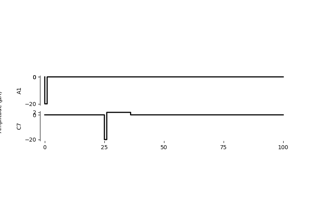Generating monophasic and biphasic pulses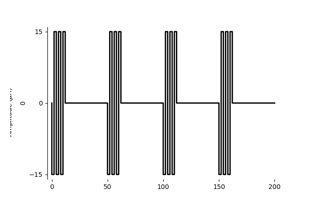Generating pulse trains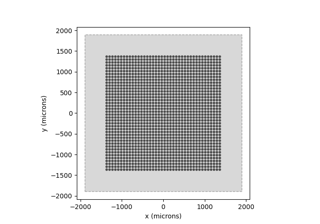Generating a stimulus from an image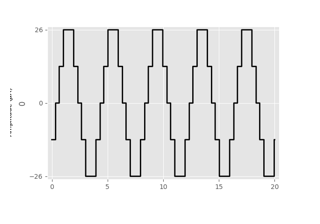Generating a sinusoidal pulse trainBeyeler et al. (2019): Axonal streaks with the axon map modelBeyeler et al. (2019): Focal percepts with the scoreboard model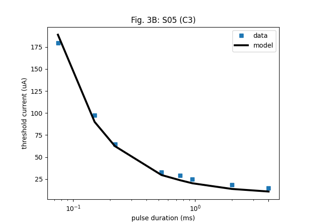Horsager et al. (2009): Predicting temporal sensitivity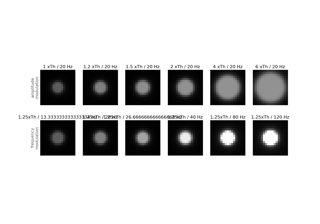Nanduri et al. (2012): Frequency vs. amplitude modulation